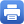Columbia Home
Dec. 11: Si Li

Title: How to count constant maps?

Abstract:

The art of using quantum field theory to derive mathematical results often lies in a mysterious transition between infinite dimensional geometry and finite dimensional geometry. In this talk we describe a general framework to study the quantum geometry of sigma-models when they are effectively localized to small fluctuations around constant maps. We illustrate how to turn the physics idea of exact semi-classical approximation into a geometric set-up in this framework, using Gauss-Manin connection. This leads to a theory of “counting constant maps” in a nontrivial way. We explain this program by a concrete example of topological quantum mechanics and show how “counting constant loops” leads to a simple proof of the algebraic index theorem. If time permits, I will explain a work in progress on a worldsheet theory of B-model, by counting constant maps from Riemann surfaces to Calabi-Yau target.

Wednesday, December 11, 4:30 – 5:30 p.m.

Mathematics 520
Tea will be served at 4:00 p.m.Print this page